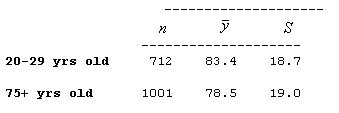## Assignment: Using Technology with Data to Run a Hypothesis Test

The purpose of this activity is to give you guided practice in carrying out the two-sample t-test, and to show you how to use software to aid in the process.

### Background

Do undergraduates sleep less than graduate students? A student conducted a study of sleep habits at a large state university. His hypothesis is that undergraduates will party more and sleep less than graduate students. He surveyed random samples of 75 undergraduate students and 50 graduate students. Subjects reported the hours they sleep in a typical night.

For this hypothesis test, he defined the population means as follows:

• μ1 is the mean number of hours undergraduate students sleep in a typical night.
• μ2 is the mean number of hours graduate students sleep in a typical night.

### Question 1:

State the null and alternative hypotheses that are being tested here.

### Question 2:

Explain why we can safely use the two-sample T-test in this case.

Comment: Before we move on to carry out the test, it is important to realize that in the two-sample problem, the data can be provided in three possible ways:

(i) Sample data in one column, and another column that indicates which sample the observation belongs to. Recall that this is the way the data were given in our leading example (looks vs. personality score and gender):Note that essentially, one column contains the explanatory variable, and one contains the response.

(ii) Sample data in different columns—data from each of the two samples appear in a column dedicated to that category. As you’ll see, this is the way the data are provided in this example:(iii) Summarized data—we are not given the actual data, but just the data summaries: sample sizes, sample means and sample standard deviations of both samples. Recall that in our second example, the data were given in this format.### Instructions

Click on the link corresponding to your statistical package to see instructions for completing the activity, and then answer the questions below.

### Question 3:

Carry out the test and report the test statistic and P-value.